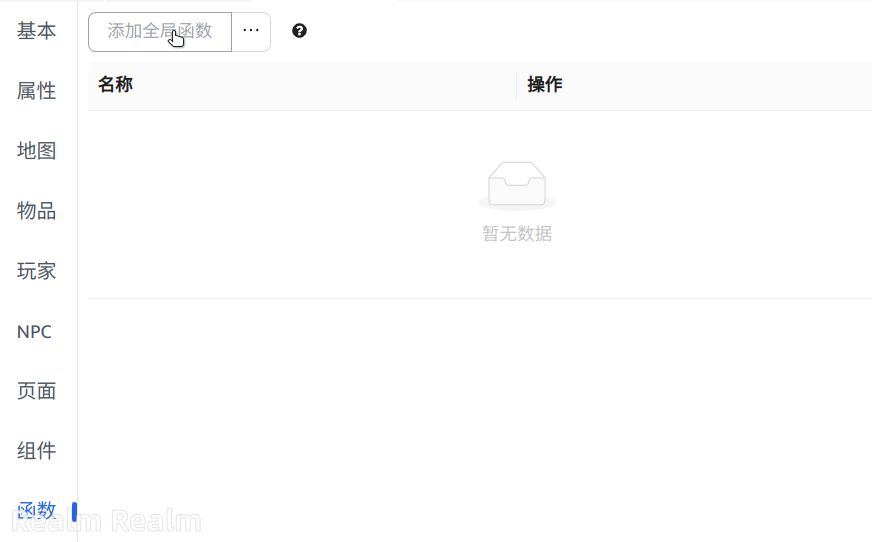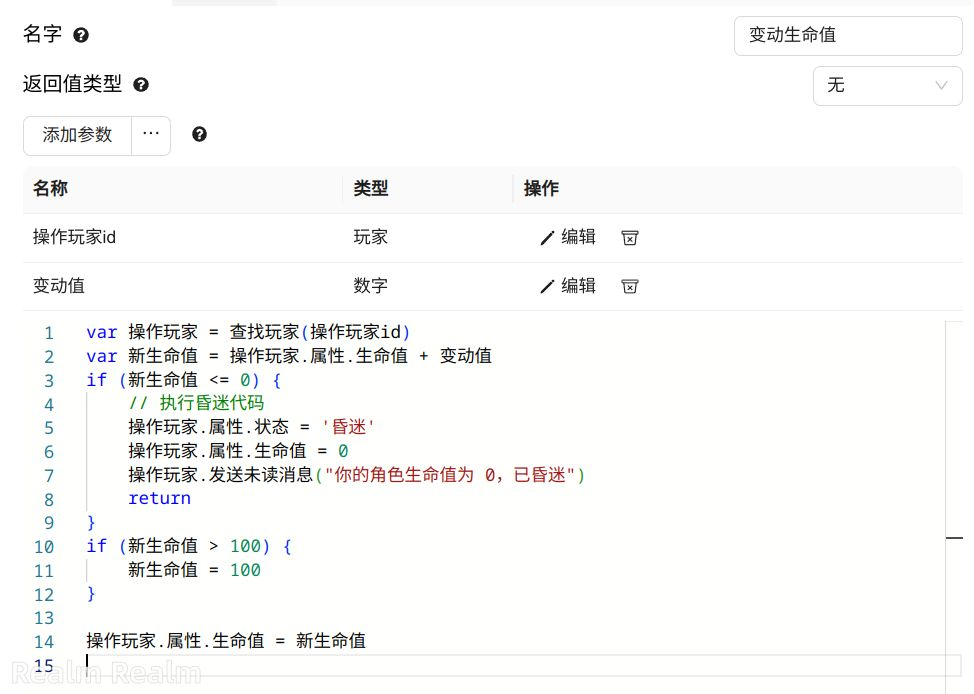# 全局函数

## 创建函数## 代码

``````属性.金钱 += 1000

``````

``````函数.赠送新手礼包() // 会进入函数代码内部
// 执行完函数后，继续执行下面的代码
``````

## 参数``````var 操作玩家 = 查找玩家(操作玩家id)
var 新生命值 = 操作玩家.属性.生命值 + 变动值
if (新生命值 <= 0) {
// 执行昏迷代码
操作玩家.属性.状态 = '昏迷'
操作玩家.属性.生命值 = 0
操作玩家.发送未读消息("你的角色生命值为 0，已昏迷")
return
}
if (新生命值 > 100) {
新生命值 = 100
}

``````

``````函数.变动生命值(玩家.id, -10)

``````

## 返回值

``````var 金钱威望 = 属性.金钱 /10
var 战斗力威望 = 属性.战斗力
var 历史战绩威望 = 属性.历史战绩 / 100
return  金钱威望 + 战斗力威望 + 历史战绩威望
``````

``````var 威望 = 函数.获得玩家威望()

``````